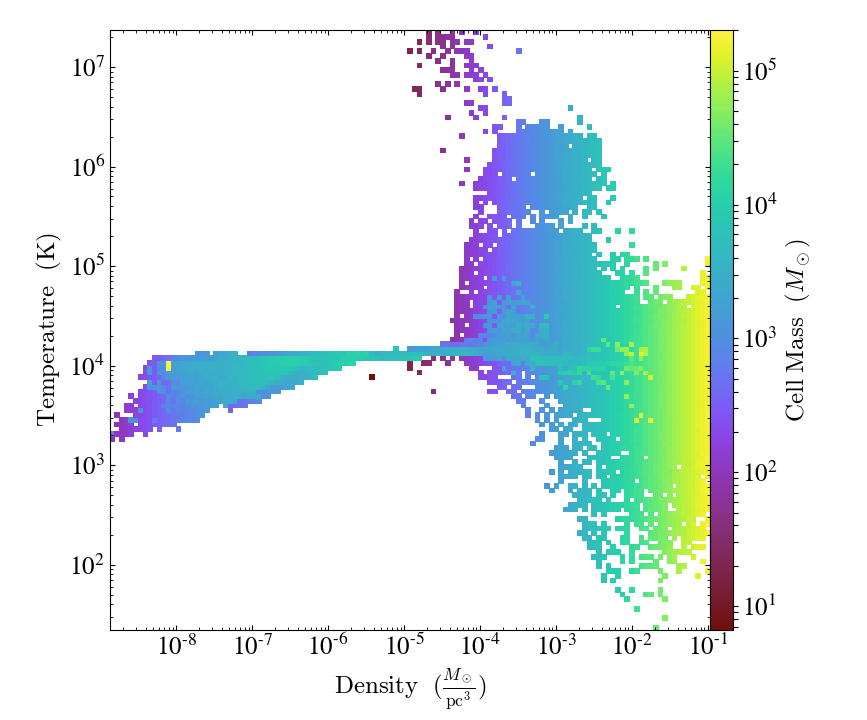# Units and Plotting¶

Notebook

It's now easy to adjust the units of a field you are plotting.

Note: the following examples use SlicePlot, but the same thing should work for ProjectionPlot, OffAxisSlicePlot, and OffAxisProjectionPlot.

First, let's create a new SlicePlot.

In :
import yt
slc = yt.SlicePlot(ds, 2, 'density', center=[0.5, 0.5, 0.5], width=(15, 'kpc'))
slc.set_figure_size(6)

/usr/lib64/python3.6/site-packages/h5py/__init__.py:34: FutureWarning: Conversion of the second argument of issubdtype from float to np.floating is deprecated. In future, it will be treated as np.float64 == np.dtype(float).type.
from ._conv import register_converters as _register_converters

Out: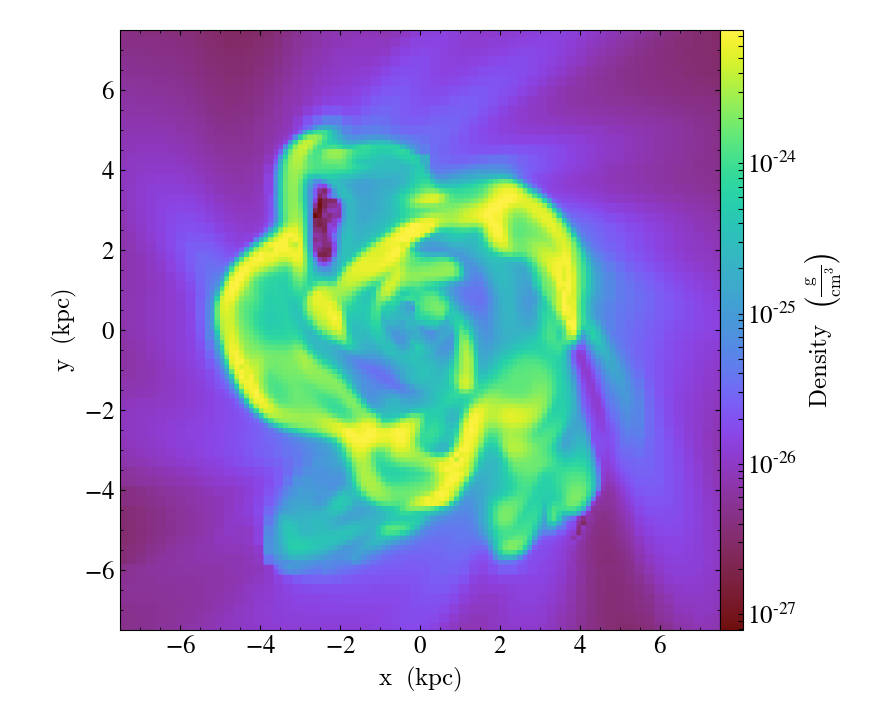The units used to scale the colorbar can be adjusted by calling the set_unit function that is attached to the plot object. This example creates a plot of density in code units:

In :
slc.set_unit('density', 'code_mass/code_length**3')

Out: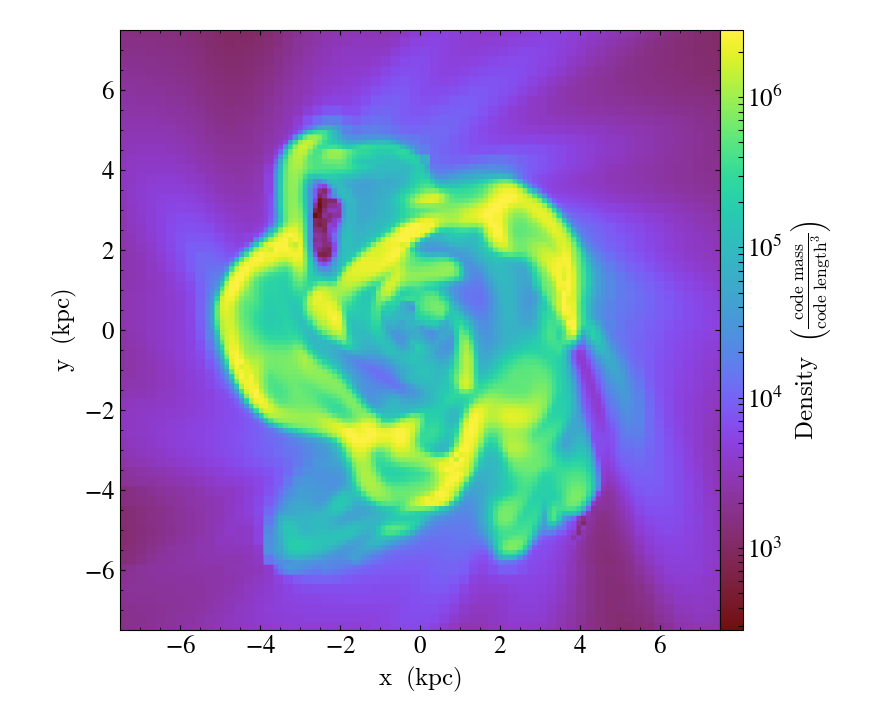This example creates a plot of gas density in solar masses per cubic parsec:

In :
slc.set_unit('density', 'Msun/pc**3')

Out: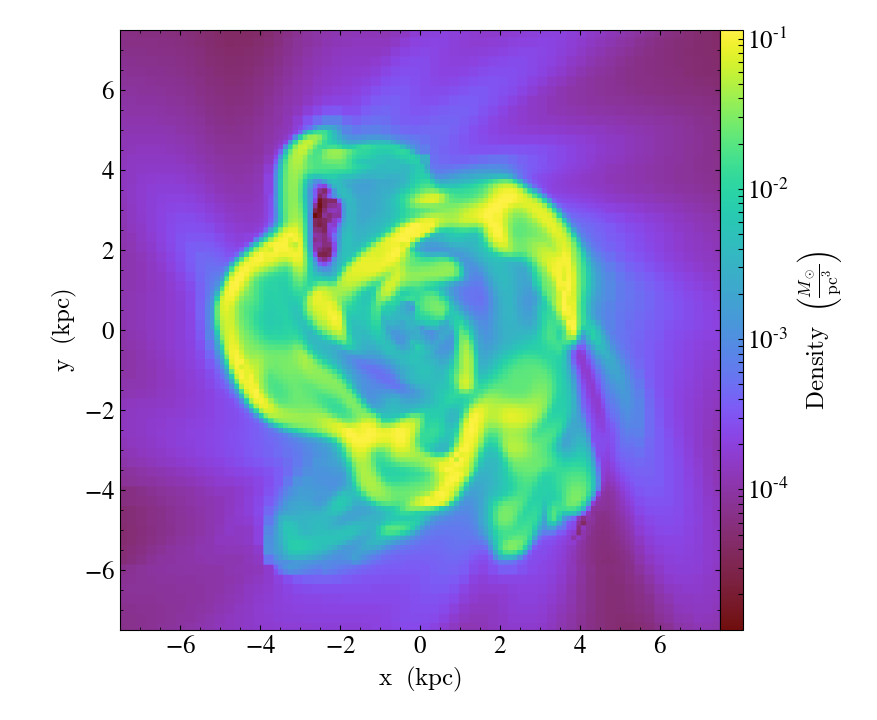The set_unit function will accept any unit string that is dimensionally equivalent to the plotted field. If it is supplied a unit that is not dimensionally equivalent, it will raise an error:

In :
from yt.utilities.exceptions import YTUnitConversionError

try:
slc.set_unit('density', 'Msun')
except YTUnitConversionError as e:
print (e)

Unit dimensionalities do not match. Tried to convert between Msun/pc**3 (dim (mass)/(length)**3) and Msun (dim (mass)).


Similarly, set_unit is defined for ProfilePlot and PhasePlot instances as well.

To illustrate this point, let's first create a new ProfilePlot:

In :
dd = ds.all_data()
plot = yt.ProfilePlot(dd, 'density', 'temperature', weight_field='cell_mass')
plot.show()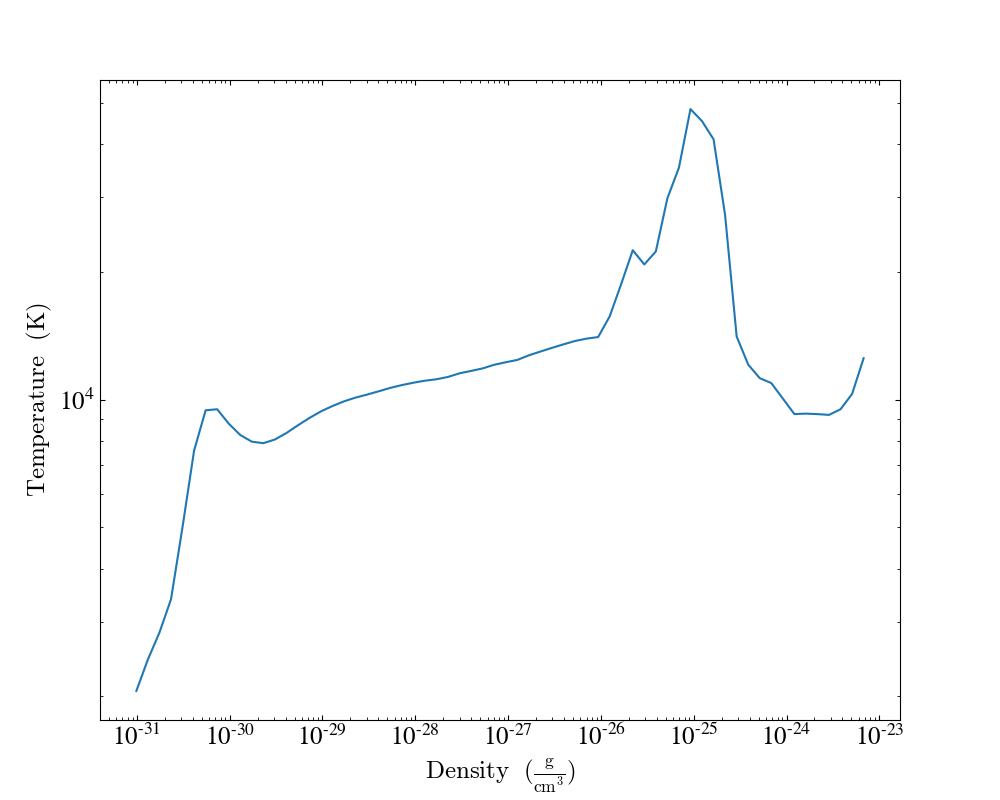And adjust the unit of the y-axis:

In :
plot.set_unit('density', 'Msun/pc**3')

Out: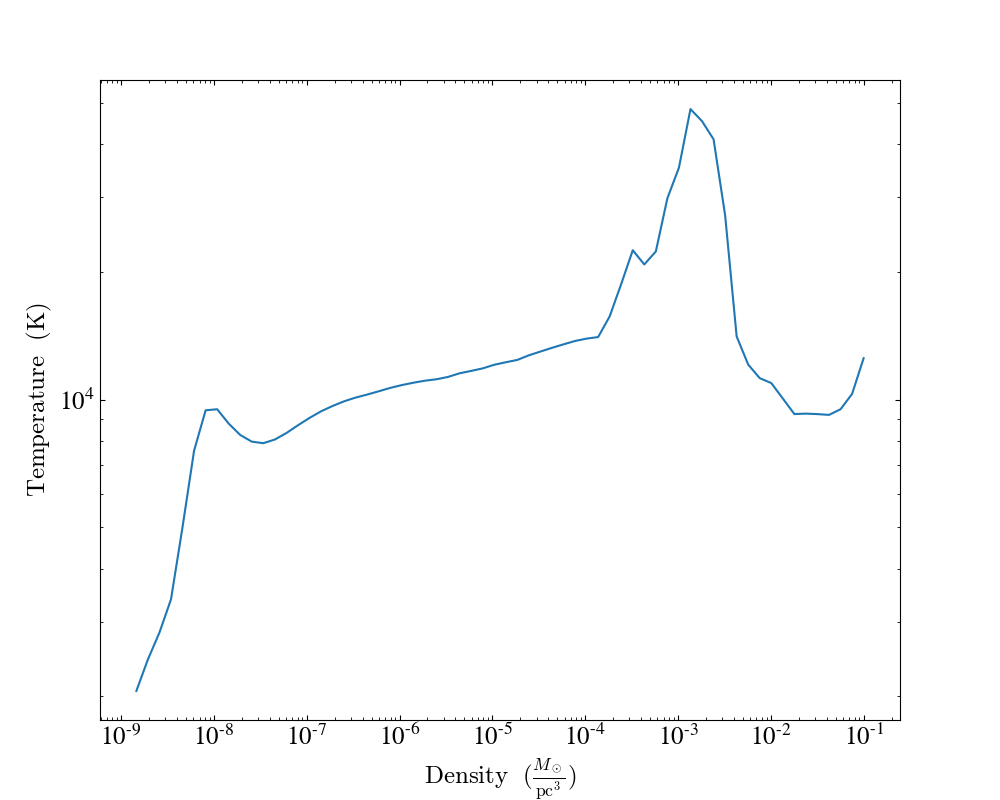Similarly for PhasePlot:

In :
plot = yt.PhasePlot(dd, 'density', 'temperature', 'cell_mass')
plot.set_figure_size(6)

Out: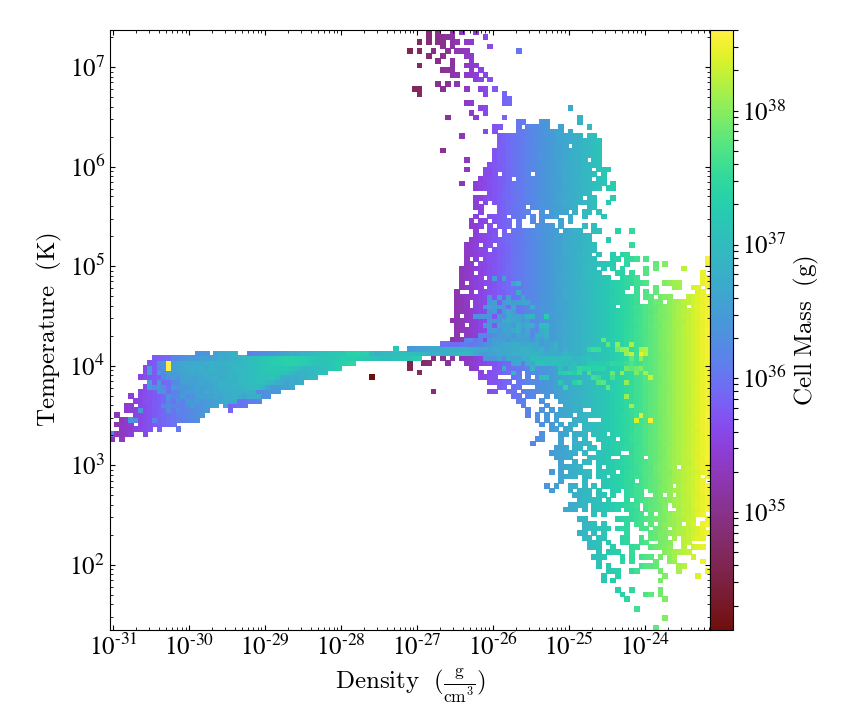In :
plot.set_unit('cell_mass', 'Msun')
plot.set_unit('density', 'Msun/pc**3')

Out: